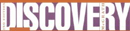Encyclopedia Article

# E, the Number - Research Article from World of Scientific Discovery

This encyclopedia article consists of approximately 2 pages of information about E, the Number.
 This section contains 364 words (approx. 2 pages at 300 words per page)

The number e has proven to be of great importance to mathematicians, with applications that include number theory, probability and statistics, biological and physical sciences, ballistics, engineering and finance. The exponential function with e as base plays a fundamental role in calculus because this function is left unchanged by the process of differentiation. The number e is an irrational number equal to 2.718282, carried out to six decimal places.

The exact birth of the number that would later by denoted by e is unknown. Its origin probably dates from the early seventeenth century in experimental observations about the effect of compounding interest over shorter and shorter time periods. When John Napier created the first table of logarithms in 1614, he used a number close to 1/e as the base, although he employed neither the concept of a base nor the notation e in his work. An appendix of the 1618 English translation of Napier's Descriptio, probably written by William Oughtred, contains the computation of the logarithm of 10 using the base e. This seems to be the first explicit use of the number e in mathematics.

The introduction of the actual letter e to represent the base of the natural logarithm is due to Leonard Euler, who first used this notation in a manuscript written in 1727 or 1728, but not published until 80 years after his death. The earliest appearance of e in a published work was in Euler's Mechanica in 1736. There is no consensus on why Euler chose the letter e, but it is unlikely he did so to represent his own name. Rather, it was probably the first letter in the alphabet that was not already in common use in mathematics. Euler's most influential publication was his Introductio in analysin infinitorum, published in 1748. In this work he demonstrated the important role that the number e and the exponential function ex has in analysis.

In 1728 Euler worked with his friend and associate Daniel Bernoulli to calculate e to twenty-six decimal places. In 1737, Euler gave a proof that e is irrational. In 1873, Charles Hermite proved that e is transcendental, that is, that e is not the root of a polynomial equation with integer coefficients.

 This section contains 364 words (approx. 2 pages at 300 words per page)
More summaries and resources for teaching or studying E, the Number.